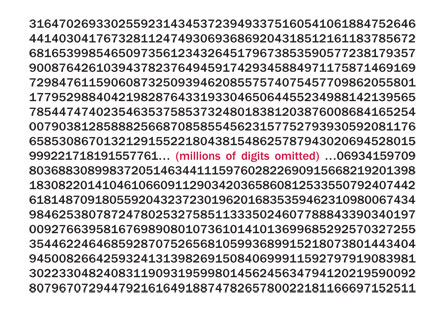Search IntMath
Close

450+ Math Lessons written by Math Professors and Teachers

5 Million+ Students Helped Each Year

1200+ Articles Written by Math Educators and Enthusiasts

Simplifying and Teaching Math for Over 23 Years

# Largest known prime: 2^43,112,609 - 1

By Murray Bourne, 22 Jun 2009

A reader wrote to say that there was an error on the page Number Properties in Interactive Mathematics. It said:

213466917 − 1. (This is huge - around 4 × 10506,756).

When I wrote the page, this was indeed the highest known prime, but in 2008, the GIMPS project (the distributed computing Great Internet Mersenne Prime Search) found an even larger one:

243,112,609 − 1

This prime has around 13 million digits. Here are some of them (with millions of digits omitted in the middle):[Image source: ScienceNews - no longer available]

It's an example of a Mersenne Prime, which are of the form 2x − 1.

The first Mersenne Prime is 22 − 1 = 3, and the next is 23 − 1 = 7.

But not all numbers in this form are prime, for example 24 − 1 = 15 is not prime.

So finding primes is a matter of trying higher values of x in the expression 2x − 1 and testing if they can be factored.

## What is a prime?

A prime has exactly 2 factors, itself and 1. So the first prime is the number 2 (factors 2 and 1).

## Who Cares?

Every time you use a password to access a bank account or web site, there is a good chance that a large prime number was used in the encryption process.

## Want to make some money with primes?

The Electronic Frontier Association paid $100,00 for the above prime, and are offering$150,000 for a prime with 100 million digits and \$250,000 for one with 1 billion digits.

This is where distributed computing power becomes important — according to ZDNet, a single computer would need 500 years to test a billion-digit prime number.

Be the first to comment below.

### Comment Preview

HTML: You can use simple tags like <b>, <a href="...">, etc.

To enter math, you can can either:

1. Use simple calculator-like input in the following format (surround your math in backticks, or qq on tablet or phone):
a^2 = sqrt(b^2 + c^2)
(See more on ASCIIMath syntax); or
2. Use simple LaTeX in the following format. Surround your math with $$ and $$.
$$\int g dx = \sqrt{\frac{a}{b}}$$
(This is standard simple LaTeX.)

NOTE: You can mix both types of math entry in your comment.